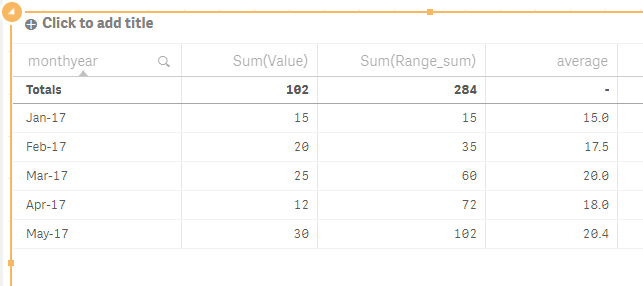# New to Qlik Sense

Discussion board where members can get started with Qlik Sense.

Announcements
Qlik® Product Spotlight: Discover what’s possible. Get more from our products.
See for yourself. Register today.
Contributor II

## How to get average of Cumilative sum values

Hi Team,

I am having numbers need to be added as cumilatively. Range sum() will do that. After that i need to get average of those numbers monthly. I am having 5 years of data.

For example

In this case if i want to calculate Avg for May-17 i need to consider (72+102)/2 insted of (12+30)/2

 monthyear Value Range sum Jan-17 15 15 Feb-17 20 35 Mar-17 25 60 Apr-17 12 72 May-17 30 102

How can i achieve this. Thanks in advance.

Regards,

Tags (1)
1 Solution

Accepted Solutions
Valued Contributor III

## Re: How to get average of Cumilative sum values

Thanks,

Arvind Patil

21 Replies
Honored Contributor III

## Re: How to get average of Cumilative sum values

like this ?Valued Contributor

## Re: How to get average of Cumilative sum values

Hi,

You want that current month avg=(last Month rangesum value + Current month rangesum value)/2

Correct?

Can you please elaborate more.

Thanks,
Neha

Contributor II

## Re: How to get average of Cumilative sum values

Hi Arul,

Not exactly,

To calculate average from januay to may- i need to consider only Sum(Range_sum)

I need an expression here, based on the date selection i need to get the average.

example for May-17 average will be (72+102)/2=87

If i select April and May 2017 average =(60+72+102)/3=78 is my desired output.

Regards,

Contributor II

## Re: How to get average of Cumilative sum values

Yes neha, based on the months selected by user need to get those month rangesum average

Valued Contributor

## Re: How to get average of Cumilative sum values

use this-

((Aggr(RangeSum(Above(Sum(Value),1,RowNo(TOTAL))),monthyear))

+

(

If(Dimensionality()=1

,Aggr(RangeSum(Above(Sum(Value),0,RowNo(TOTAL))),monthyear)

,IF(Dimensionality()=0

,Sum(Value)

,RangeSum(Above(Total Sum(Value),0,RowNo(TOTAL))))))

)

/

2

Contributor II

## Re: How to get average of Cumilative sum values

Hi Neha,

Thanks for your time.

It's not working for me. I am not considering only one month here. even for one month also formula is not working.

Will it only work for time dimension or works for all the dimensions?

Regards,

Valued Contributor

## Re: How to get average of Cumilative sum values

Hi,

It should work for no any selection of month. See the attached file.

You need month to be changes according to your selection.

Thanks ,

Neha

Contributor II

## Re: How to get average of Cumilative sum values

Can you share QVF file. That will help me. I am using sense server.

Valued Contributor

## Re: How to get average of Cumilative sum values

ohh...& i'm using Qlikview